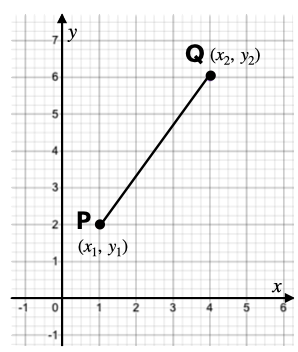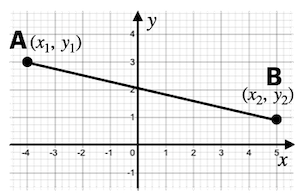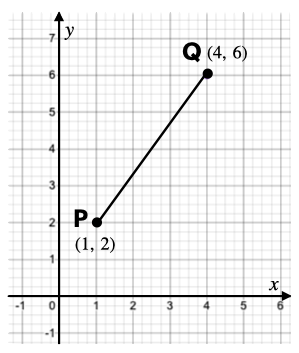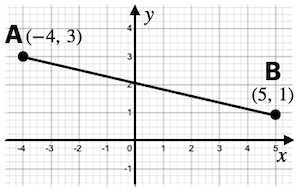# High School Geometry - Distance and Midpoint Formulas

## Distance Formula

• If the coordinates of any two points are known, then the distance between the points can easily be found using the distance formula.
• Consider two points $P\left({x}_{1},{y}_{1}\right)$ and $Q\left({x}_{2},{y}_{2}\right)$ as shown on the graph below.• The formula to find the distance between the points P and Q is:

$\left|PQ\right|=\sqrt{{\left(∆abscissa\right)}^{2}+{\left(∆ordinate\right)}^{2}}$

, or

• This formula can be derived by using Pythagorean theorem.

## Midpoint Formula

• If the coordinates of the endpoints of a line segment are known, then the coordinates of its midpoint can easily be found using the midpoint formula.
• Consider a line segment AB as shown in the figure.• Let M be the mid-point of AB, then the coordinates of M are given by the formula:

## Solved Examples

Question 1: Find the distance between the points P and Q shown in the graph.Solution: It is given that P(1, 2) & Q(4, 6).

$\left|PQ\right|=\sqrt{{\left({x}_{2}-{x}_{1}\right)}^{2}+{\left({y}_{2}-{y}_{1}\right)}^{2}}$

$=\sqrt{{\left(4-1\right)}^{2}+{\left(6-2\right)}^{2}}$

$=\sqrt{9+16}=\sqrt{25}=5$

Question 2: Find the midpoint of the line segment AB shown in the graph.Solution: It is given that A(-4, 3) & B(5, 1).

Let  be the coordinate of the midpoint of AB.

$x=\frac{{x}_{1}+{x}_{2}}{2}=\frac{-4+5}{2}=\frac{1}{2}$

$y=\frac{{y}_{1}+{y}_{2}}{2}=\frac{3+1}{2}=2$

Hence, the midpoint of segment AB is .

## Cheat Sheet

• The distance between the points  and  is given by:

$\left|PQ\right|=\sqrt{{\left({x}_{2}-{x}_{1}\right)}^{2}+{\left({y}_{2}-{y}_{1}\right)}^{2}}$

• The coordinates of the midpoint of a line segment AB where  and  are given by:

## Blunder Areas

• One must be careful while substituting the values with a negative sign.